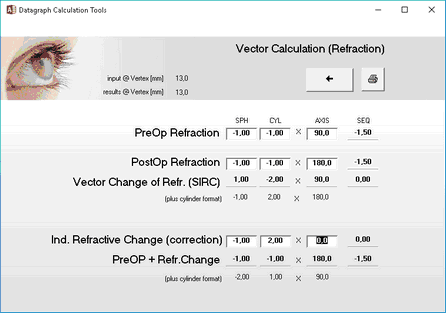﻿ Tools and Default Settings > Vector Calculator (Refraction)
 Navigation:  Tools and Default Settings > Vector Calculator (Refraction)The Vector Calculation tool allows you to calculate the vectorial change of refraction between a pre and postOP refraction or calculate the postOP refraction for a given induced refractive change. Plus and minus cylinder notation is acceptable and different vertex distance values can be used. (Setting of the vertex distance for results is done here by changing the value for 'used for Graphs and Nomograms')